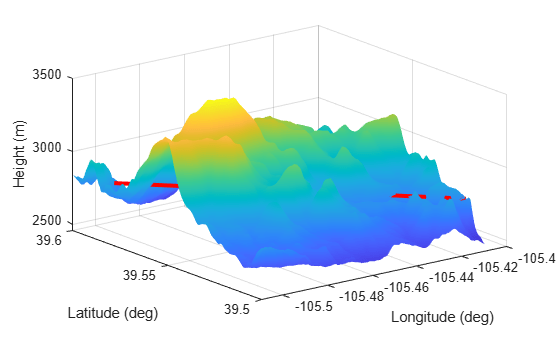# occlusion

Test for occlusion of point by a surface

## Syntax

``status = occlusion(surface,point1,point2)``

## Description

example

````status = occlusion(surface,point1,point2)` returns `status` as `true` if the line-of-sight between `point1` and `point2` on a surface is occluded by parts of the `surface`.```

## Examples

collapse all

Create a radar scenario and specify set the `IsEarthCentered` property as true to obtain the terrain from a DTED file.

`scene = radarScenario(IsEarthCentered = true);`

Model the reflectivity as a constant gamma surface.

`refl = surfaceReflectivityLand(Model = 'ConstantGamma',Gamma = -20);`

Add a 0.1-by-0.1 degree land surface derived from a DTED file.

```bdry = [39.5 39.6;-105.51 -105.41]; srf = landSurface(scene,Terrain = 'n39_w106_3arc_v2.dt1', ... Boundary = bdry,RadarReflectivity = refl);```

Verify that occlusion is turned on.

`mgr = scene.SurfaceManager`
```mgr = SurfaceManager with properties: UseOcclusion: 1 Surfaces: [1x1 radar.scenario.LandSurface] ```

Plot the surface height.

```x = linspace(srf.Boundary(2,1),srf.Boundary(2,2),201); y = linspace(srf.Boundary(1,1),srf.Boundary(1,2),201); [X,Y] = meshgrid(x,y); X1 = X(:)'; Y1 = Y(:)'; H = height(srf,[Y1;X1]); H = reshape(H,length(x),length(y)); surf(x,y,H) shading interp ylabel('Latitude (deg)') xlabel('Longitude (deg)') zlabel('Height (m)') hold on```

Test for occlusion.

`ht1 = height(srf,[39.59 -105.5])`
```ht1 = 2810 ```
`ht2 = height(srf,[39.51 -105.41])`
```ht2 = 2786 ```
`occlusion(srf,[39.59 -105.5 ht1],[39.51 -105.41 ht2])`
```ans = logical 1 ```

The points are occluded. The line between the two points passes through the surface as shown.

`plot3([-105.5 -105.41],[39.59 39.51], [ht1 ht2],'r','LineWidth',3)`Create a square sea surface assuming a moderate sea state with a wind speed of about 12 knots (6.17 m/s), a fetch of 120 nmi (222.24 km), and a length of 1.024 km. Add an Elfouhaily spectrum to the sea surface. Use the `occlusion` object function to determine if the path from point 1 to point 2 is occluded.

Start by creating a radar scenario;

`scene = radarScenario;`

Add a sea surface with an Elfouhaily spectrum.

```rng('default'); spec = seaSpectrum('Resolution',16);```

Create the sea surface.

```bnds = [0 1024; 0 1024]; srf = seaSurface(scene,'Boundary',bnds, ... 'WindSpeed',6.17,'Fetch',222.24e3, ... 'SpectralModel',spec);```

Set two points for testing occlusion.

```p1 = [1016; 368; -0.082]; p2 = [10; 100; 0.13];```

Determine if the path from p1 to p2 is occluded

`tf1 = occlusion(srf,p1,p2)`
```tf1 = logical 1 ```

## Input Arguments

collapse all

Surface, specified as a `LandSurface` object or `SeaSurface` object.

Position of the first point on a surface, specified as a length-3 real-valued vector.

If the `IsEarthCentered` property of the `radarScenario` object is specified as:

• `false` — Specify the three elements as the x-, y-, and z-coordinates in the reference frame of the tracking scenario. Units are in meters,

• `true` — Specify the three elements as the latitude in degrees, longitude in degrees, and altitude in meters, in the geodetic frame.

Data Types: `double`

Position of the second point on a surface, specified as a length-3 real-valued vector.

If the `IsEarthCentered` property of the `radarScenario` object is specified as:

• `false` — Specify the three elements as the x-, y-, and z-coordinates in the reference frame of the tracking scenario. Units are in meters,

• `true` — Specify the three elements as the latitude in degrees, longitude in degrees, and altitude in meters, in the geodetic frame.

Data Types: `double`

## Output Arguments

collapse all

Occlusion status, returned as a logical `1` (`true`) representing occluded, or `0` (`false`) representing not occluded.

## Version History

Introduced in R2022a